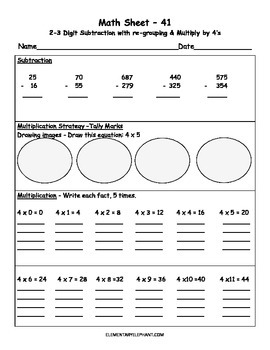# MATH Skill Sheets & Mini-Lessons MONTH 5 - Multiplication # 4,5,6,7Subject
Resource Type
File Type

PDF

(947 KB|20 pages)
Standards
• Product Description
• StandardsNEW
In this Set for Month 5, this is what you get:
MATH Practice Sheets #41-49 and 4 Weeks of Mini-Lesson Plans

Week 1 Contents
Sheet 40 – 4’s Multiplication Facts, 5 x’s each
Sheet 41 - 2-3 Digit Subtraction with re-grouping & multiply by 4’s, using tally marks strategy
Sheet 42 – Multiply by 4’s Quiz

Week 2 Contents
Sheet 43 - Math Multiplication Review (2, 3 and 4’s)
Sheet 44 – 5’s Multiplication Facts, 5x’s each
Sheet 45 – 5’s Multiplication Quiz

Week 3 Contents
Sheet 46 – 6’s Multiplication Facts, 5x’s each
Sheet 47 – 6’s Multiplication Facts Quiz

Week 4 Contents
Sheet 48 – 7’s Multiplication Facts, 5x’s each
Sheet 49 – 7’s Multiplication Facts Quiz
COMPREHENSIVE Test – Multiplication Facts 5, 6, 7’s

How does the Elementary Elephant Math Program work?
This is a spiral Math curriculum that can be used in various ways. You can easily use the Math sheets as worksheets, however, they are more than that! Some teachers have used this program to review skills during Summer School!

Suggested use:
Use as outlined in the provided lesson plans, in a monthly format. Please see the lesson plans that I have created for ideal use of this program. Be sure to view each set and focus skills, as each will be repeated again in consecutive Math Sets.

Who is this for?
3rd, 4th and 5th Grade students. These are great for students on (RTI) Response to Intervention, (PST) the Problem Solving Team require repeated practice in various ways and in smaller chunks of learning spread out over time. I created and used this program for my 4th and 5th grade Resource Math students as a replacement Math Curriculum. I was inspired by research conducted by the University of Chicago, based on Spiral Math. Read more at: http://everydaymath.uchicago.edu/about/why-it-works/spiral/
Interpret products of whole numbers, e.g., interpret 5 × 7 as the total number of objects in 5 groups of 7 objects each. For example, describe a context in which a total number of objects can be expressed as 5 × 7.
Fluently multiply multi-digit whole numbers using the standard algorithm.
Fluently add and subtract multi-digit whole numbers using the standard algorithm.
Multiply one-digit whole numbers by multiples of 10 in the range 10–90 (e.g., 9 × 80, 5 × 60) using strategies based on place value and properties of operations.
Fluently add and subtract within 100 using strategies based on place value, properties of operations, and/or the relationship between addition and subtraction.
Total Pages
20 pages
Not Included
Teaching Duration
1 month
Report this Resource to TpT
Reported resources will be reviewed by our team. Report this resource to let us know if this resource violates TpT’s content guidelines.\$5.99
List Price:
\$6.99
You Save:
\$1.00